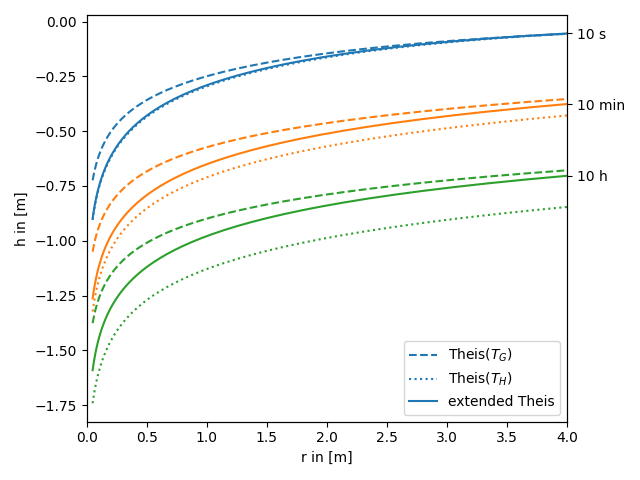# The extended Theis solution in 2D

We provide an extended theis solution, that incorporates the effectes of a heterogeneous transmissivity field on a pumping test.

In the following this extended solution is compared to the standard theis solution for well flow. You can nicely see, that the extended solution represents a transition between the theis solutions for the geometric- and harmonic-mean transmissivity.

Reference: Zech et. al. 2016

```import numpy as np
from matplotlib import pyplot as plt

from anaflow import ext_theis_2d, theis
```

We use three time steps: 10s, 10min, 10h

```time_labels = ["10 s", "10 min", "10 h"]
time = [10, 600, 36000]  # 10s, 10min, 10h
```

Radius from the pumping well should be in [0, 4].

```rad = np.geomspace(0.05, 4)
```

Parameters of heterogeneity, storage and pumping rate.

```var = 0.5  # variance of the log-transmissivity
len_scale = 10.0  # correlation length of the log-transmissivity
TG = 1e-4  # the geometric mean of the transmissivity
TH = TG * np.exp(-var / 2.0)  # the harmonic mean of the transmissivity

S = 1e-4  # storativity
rate = -1e-4  # pumping rate
```

Now let’s compare the extended Theis solution to the classical solutions for the near and far field values of transmissivity.

```head_TG = theis(time, rad, S, TG, rate)
time_ticks = []
for i, step in enumerate(time):
label_TG = "Theis(\$T_G\$)" if i == 0 else None
label_TH = "Theis(\$T_H\$)" if i == 0 else None
label_ef = "extended Theis" if i == 0 else None
plt.plot(
)
plt.plot(
)

plt.xlabel("r in [m]")
plt.ylabel("h in [m]")
plt.legend()
ylim = plt.gca().get_ylim()